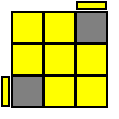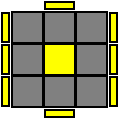169 Pages

Algorithms (algs for short) are a set of moves that are done in a specific order to achieve a specific end result in orienting or permuting the pieces on a Rubik's Cube. A special notation is used to describe which face needs to be rotated in which direction. A list of algorithm notations are listed below.

## Notations

### One face/layer - F, U, R, B, D, and L

F - Front face clockwise

F' - Front face counter-clockwise

U - Up face clockwise

U' - Up face counter-clockwise

R - Right face clockwise

R' - Right face counter-clockwise

B - Back face clockwise

B' - Back face counter-clockwise

D - Down face clockwise

D' - Down face counter-clockwise

L - Left face clockwise

L' - Left face counter-clockwise

F2 - Front face rotate 180°

U2 - Up face rotate 180°

R2 - Right face rotate 180°

B2 - Back face rotate 180°

D2 - Down face rotate 180°

L2 - Left face rotate 180°

### Two layers - w, f, u, r, b, d, and l

w/w' - Adds on to a letter (example: Fw) to indicate to turn two layers

f - Front face double layer clockwise

f' - Front face double layer counter-clockwise

u - Up face double layer clockwise

u' - Up face double layer counter-clockwise

r - Right face double layer clockwise

r' - Right face double layer counter-clockwise

b - Back face double layer clockwise

b' - Back face double layer counter-clockwise

d - Down face double layer clockwise

d' - Down face double layer counter-clockwise

l - Left face double layer clockwise

l' - Left face double layer counter-clockwise

f2 - Front face double layer rotate 180°

u2 - Up face double layer rotate 360 degrees

r2 - Right face double layer rotate 180°

b2 - Back face double layer rotate 180°

d2 - Down face double layer rotate 180°

l2 - Left face double layer rotate 180°

### Layer slices - M, E, and S

M - Turn the layer between L face and R face down (in the direction of an L move)

M2- Turn the layer between L face and R face twice

M' - Turn the layer between L face and R face up (in the direction of an R move)

E - Turn the layer between U and D face counter-clockwise (in the direction of a D move)

E' - Turn the layer between U and D face clockwise (in the direction of a U move)

S - Turn the layer between the F and B face clockwise (in direction of an F move)

S' - Turn the layer between the F and B face counter-clockwise (in direction of a B move)

### Entire cube orientation - x, y, and z

x - Turn the cube to R/L' direction

x' - Turn the cube in direction of L/R'

y - Turn the cube in direction of U

y' - Turn the cube in direction of D/U'

z - Turn the cube in direction of F

z' - Turn the cube in direction of B/F'

## OLLF' RW U R' U' RW' F R(R U2 R') (R' F R F') U2 (R' F R F')F R U R' U' F' f R U R' U' f'Fw (R U R' U') Fw' U' F (R U R' U') F'Fw (R U R' U') Fw' U F (R U R' U') F'(y2) r' R U R U R' U' r R2' F R F'F R U R' d R' U2 R' F R F'Fw R U R' U' Fw' U R U R' U' R' F R F'M' U R U R' U' M2 U R U' Rw'F R U R' U' F'R U R' U' R' F R F'R' U' R' F R F' U R(R U R2' U') (R' F) (R U) (R U') F'(y) r U2 R' U' R U' r'(y) l' U2 L U L' U l(y) r U R' U R U2 r'(y) l' U' L U' L' U2' lr' U' R U' l U' R' U l' U2' rr U R' U l' U R U' l U2 r'R' F R U R' U' F' U R(y2) L F' L' U' L U F U' L'R' U2 R2 U R' U R U2 (x') U' R' UR' U' R U' R' d R' U R B(y) f R U R' U' R U R' U' f'(y) Fw (R U R' U') Fw' F (R U R' U') (R U R' U') F'R d L' d' R' U R B R'R' d' L d R U' R' F' Ry' Fw R U R' U' Fw'y Fw' L' U' L U FwF R U R' U' R U R' U' F'F' L' U' L U L' U' L U Fl' U R' U' R l (U2 x') U' R U l'l U' R U R' l' U2 F R' F' Rr' U' R U' R' U R U' R' U2 rr U R' U R U' R' U R U2' r'(y) R U R' U R U' R' U' R' F R F'R' U' R U' R' U R U l U' R' UR' U' R (y'x') R U' R' F R U l'R U R' (xz') R' U R B' R' U' lR' U2 l R U' R' U l' U2' R(y) F R U' R' U' R U R' F'R' F R U l' U' l (y') R U' R'(x') R U' R' F' R U R' (x y) R' U R(Rw U Rw') (R U R' U') (Rw U' Rw')y2 (Lw' U' Lw) (L' U' L U) (Lw' U Lw)B' R B' R2' U R U R' U' R B2R2' U R' B' R U' R2' U l U l'R U R' U R U2 R' F R U R' U' F'y' (R' F R F') (R' F R F') (RU R' U') (R U R' U') FASTER ALGO(R U R' U') (M' U R U') Rw'y2 (M' U M) U2 (M' U M)

## PLL

The PLL algorithms finishes the cube by permutating the last layer. All of the last layer pieces are moved to their proper locations. The red arrows show movements of the edge pieces, and the blue arrows show the movements of the corner pieces.R2' U R U R' U' R' U' R' U R'y2 R U' R U R U R U' R' U' R2M2 U M2 U M U2 M2 U2 M U2M2 U(‘) M2 U2(‘) M2 U(‘) M2(x) R' U R' D2 R U' R' D2 R2 (x')(x) R2 D2 R U R' D2 R U' R (x')x’ R U’ R’ D R U R’ D’ R U R’ D R U’ R’ D’y’ R U’ R’ U’ R U R D R’ U’ R D’ R’ U2 R’R' U2 R U2 R' F R U R' U' R' F' R2 U'R U R' F' R U R' U' R' F R2 U' R' U'x R2 F R F’ R U2 r’ U r U2R U R’ U R U R’ F’ R U R’ U’ R’ F R2 U’ R’ U2 R U’ R’R’ U R U’ R’ F’ U’ F R U R’ F R’ F’ R U’ RR’ U’ F’ R U R’ U’ R’ F R2 U’ R’ U’ R U R’ U RR U R' U' R' F R2 U' R' U' R U R' F'R' U R' U' (y) R' F' R2 U' R' U R' F R FF R U' R' U' R U R' F' R U R' U' l' U R U' (x')R U R’ U’ D R2 U’ R U’ R’ U R’ U R2 D’R2 U R’ U R’ U’ R U’ R2 U’ D R’ U R DR2 U’ R U’ R U R’ U R2 U D’ R U' R’ Dy2 R' U' R U D' R2 U R’ U R U’ R U’ R2 D
Community content is available under CC-BY-SA unless otherwise noted.